# A/B TEST & CONVERSION RATE PROJECT# Analyze and Present A/B Test Results¶

This research was conducted in order to answer the questions asked for a job interview.

Facebook recently introduced a new bidding type, "average bidding”, as an alternative to its exisiting bidding type, called maximum bidding”.

One of our clients, bombabomba.com, has decided to test this new feature and wants to conduct an A/B test to understand if average bidding brings more conversions than maximum bidding.

In this A/B test, bombabomba.com randomly splits its audience into two equally sized groups, e.g. the test and the control group.

A Facebook ad campaign with “maximum bidding” is served to “control group” and another campaign with “average bidding” is served to the “test group”.

The A/B test run for 1 month and bombabomba.com now expects you to analyze and present the results of this A/B test.

You should answer the following questions in your presentation:

1. How would you define the hypothesis of this A/B test?
2. Can we conclude statistically significant results?
3. Which statistical test did you use, and why ?
4. Based on your answer to Question 2, what would be your recommendation to client?

# 📙 Load Libraries¶

In :
%%html
<style>
@import url('https://fonts.googleapis.com/css?family=Orbitron|Roboto');
a {color: #37c9e1; font-family: 'Roboto';}
h1 {color: #C20E69; font-family: 'Poppins'}
h2, h3 {color: #25B89B; font-family: 'Poppins';}
h4 {color: #818286; font-family: 'Roboto';}

</style>

In :
# Libraries

# Main
#----------------------
import pandas as pd
import numpy as np
from abtest import *

# Data Viz
#----------------------
import seaborn as sns
import matplotlib.pyplot as plt
import pylab
plt.style.use('fivethirtyeight')

# Stats
#----------------------
from scipy import stats
from scipy.stats import skewtest
from scipy.stats import median_test
from scipy.stats import shapiro
from statsmodels.stats.proportion import proportions_ztest

# Settings
#----------------------
pd.set_option("display.max_columns",None);
pd.set_option("display.max_rows",None);


# 📖 Read Data¶

In :
control = pd.read_excel(r"C:\Users\oe\VBO\datasets\ab_testing_data.xlsx", sheet_name="Control Group")
test = pd.read_excel(r"C:\Users\oe\VBO\datasets\ab_testing_data.xlsx", sheet_name="Test Group")


## 🔎 Descriptive Statistics¶

In :
def descriptive_statistics(dataframe):
print("Data Descriptive Statistics","\n")
name =[x for x in globals() if globals()[x] is dataframe]
print("Dataframe: %s" % name,"\n")
print("Data Shape: ",dataframe.shape,"\n")
print(dataframe.info(),"\n")
print("First 3 rows:","\n",dataframe.head(3),"\n")
if dataframe.isna().any().sum() == 0:
print("There are no missing value.")
else:
print(dataframe.isna().any().sum())
print("_"*60)

In :
for i in [control,test]:
print()
descriptive_statistics(i)

Data Descriptive Statistics

Dataframe: control

Data Shape:  (40, 4)

<class 'pandas.core.frame.DataFrame'>
RangeIndex: 40 entries, 0 to 39
Data columns (total 4 columns):
#   Column      Non-Null Count  Dtype
---  ------      --------------  -----
0   Impression  40 non-null     float64
1   Click       40 non-null     float64
2   Purchase    40 non-null     float64
3   Earning     40 non-null     float64
dtypes: float64(4)
memory usage: 1.4 KB
None

First 3 rows:
Impression        Click    Purchase      Earning
0  82529.459271  6090.077317  665.211255  2311.277143
1  98050.451926  3382.861786  315.084895  1742.806855
2  82696.023549  4167.965750  458.083738  1797.827447

There are no missing value.
____________________________________________________________

Data Descriptive Statistics

Dataframe: test

Data Shape:  (40, 4)

<class 'pandas.core.frame.DataFrame'>
RangeIndex: 40 entries, 0 to 39
Data columns (total 4 columns):
#   Column      Non-Null Count  Dtype
---  ------      --------------  -----
0   Impression  40 non-null     float64
1   Click       40 non-null     float64
2   Purchase    40 non-null     float64
3   Earning     40 non-null     float64
dtypes: float64(4)
memory usage: 1.4 KB
None

First 3 rows:
Impression        Click    Purchase      Earning
0  120103.503796  3216.547958  702.160346  1939.611243
1  134775.943363  3635.082422  834.054286  2929.405820
2  107806.620788  3057.143560  422.934258  2526.244877

There are no missing value.
____________________________________________________________


In this research, there are two different groups and will investigate whether there is a statistically significant difference between the averages of these two different groups.

We have 40 observation and 4 feature in this dataset.

Two different groups will be taken from the "Purchase" column for comparison.

Control group is standart bidding type: " Maximum Bidding "

Test Group is new bidding type "Average Bidding "

In :
control.describe([0.10, 0.25, 0.50, 0.75, 0.90, 0.95, 0.99]).T

Out:
count mean std min 10% 25% 50% 75% 90% 95% 99% max
Impression 40.0 101711.449068 20302.157862 45475.942965 81711.120315 85726.690349 99790.701078 115212.816543 129438.442526 132950.532455 143105.791105 147539.336329
Click 40.0 5100.657373 1329.985498 2189.753157 3582.086578 4124.304129 5001.220602 5923.803596 7010.163109 7374.361202 7761.795112 7959.125069
Purchase 40.0 550.894059 134.108201 267.028943 381.992696 470.095533 531.206307 637.957088 740.182038 748.270761 790.187785 801.795020
Earning 40.0 1908.568300 302.917783 1253.989525 1569.055776 1685.847205 1975.160522 2119.802784 2254.805009 2318.528498 2481.308737 2497.295218
In :
test.describe([0.10, 0.25, 0.50, 0.75, 0.90, 0.95, 0.99]).T

Out:
count mean std min 10% 25% 50% 75% 90% 95% 99% max
Impression 40.0 120512.411758 18807.448712 79033.834921 97389.766706 112691.970770 119291.300775 132050.578933 141739.315249 153178.691063 158245.263797 158605.920483
Click 40.0 3967.549761 923.095073 1836.629861 2917.265312 3376.819024 3931.359804 4660.497911 5074.011379 5271.186906 6012.877296 6019.695079
Purchase 40.0 582.106097 161.152513 311.629515 381.054983 444.626828 551.355732 699.862360 823.745360 854.208945 876.576104 889.910460
Earning 40.0 2514.890733 282.730852 1939.611243 2186.392257 2280.537426 2544.666107 2761.545405 2813.610925 2931.311449 3091.940894 3171.489708

Apparently, there are no outliers in these two group data, and the mean and median values are close to each other. However, box-plot graph will be applied to make sure.

## ◧ Separating Purchase columns from datasets¶

In :
AB = pd.DataFrame()

AB["A"] = control["Purchase"]
AB["B"] = test["Purchase"]
AB.describe()

Out:
A B
count 40.000000 40.000000
mean 550.894059 582.106097
std 134.108201 161.152513
min 267.028943 311.629515
25% 470.095533 444.626828
50% 531.206307 551.355732
75% 637.957088 699.862360
max 801.795020 889.910460
In :
AB.describe().T

Out:
count mean std min 25% 50% 75% max
A 40.0 550.894059 134.108201 267.028943 470.095533 531.206307 637.957088 801.79502
B 40.0 582.106097 161.152513 311.629515 444.626828 551.355732 699.862360 889.91046
In :
vals, names, xs = [],[],[]
for i, col in enumerate(AB.columns):
vals.append(AB[col].values)
names.append(col)
xs.append(np.random.normal(i + 1, 0.04, AB[col].values.shape))  # adds jitter to the data points - can be adjusted

plt.boxplot(vals, labels=names)
palette = ['#25B89B', '#C20E69']
for x, val, c in zip(xs, vals, palette):
plt.scatter(x, val, alpha=0.7, color=c)
plt.show()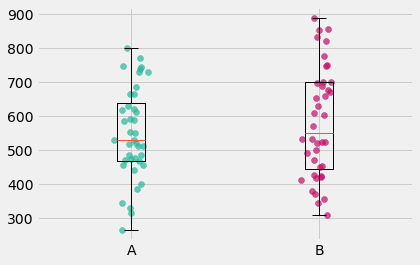A is control group -- Maximum Bidding
B is test group -- Avarage Bidding

When we looking at the averages of these two groups, the test group "average bidding", looks better.

However, this is only a mathematical inference and no business decision shouldn't be made based on this result.

Whether the result is random or not should be statistically investigated.

The main question is;

Is there a statistically significant difference between these two average of groups?

# 🔎Two Independent Sample T Test¶

Assumptions:

1. Normality Assumption
• This situation will be observed first with qqplot, then final decision will be reached with shapiro-wilks test and skewtest
2. Homogeneity of variance
• Levene test

## Q - Q Plot¶

### Control Group - Maximum Bidding¶

In :
stats.probplot(AB["A"], dist="norm", plot=pylab)
pylab.title("Control Group - Maximum Bidding")
pylab.show()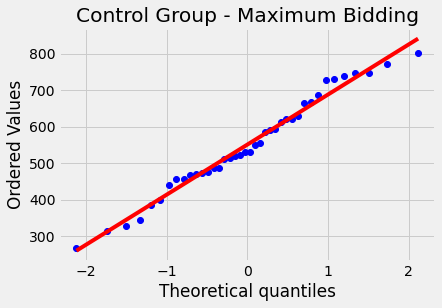The distribution seems normal, however the shapiro-wilk test will be applied to be sure.

### Test Group - Average Bidding¶

In :
stats.probplot(AB["B"], dist="norm", plot=pylab)
pylab.title("Test Group - Average Bidding")
pylab.show()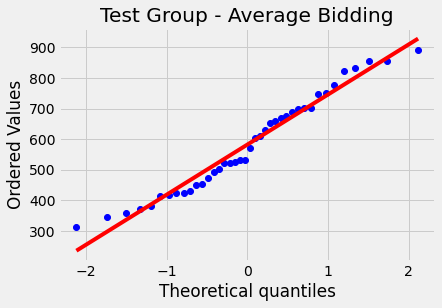## 1. Normality Assumption¶

### Shapiro - Wilk Test¶

➠ Ho: The data follows a normal distribution.

➠ H1: The data does not follow a normal distribution.

## 2. Variance Homogenity Assumption¶

### Levene Test¶

➠ Ho: There is no difference between the variance of the both groups.
➠ H1: There is a significant difference between the variance of the both groups.

## Two Independent Sample T Test Hypothesis¶

➠ Ho: There is not a statistically significant difference between the means with
➠ H1: There is a statistically significant difference between the means with

## Other Situations¶

#### At least one group does not follow a normal distribution¶

• In this case the non parametric test "Mann Whitney - U test" is applied.

#### Groups follow a normal distribution but variances are NOT homogenous¶

• In this case "Welch Test" is applied.
• For this option in Two Independent Sample T Test Function equal_var = True argument is applied.

# 📊 TEST ANALYSIS REPORT¶

In :
test_ab(AB,"A", "B", alpha = 0.05, plot=True)

⁝⁝⁝⁝⁝⁝⁝⁝⁝⁝⁝⁝⁝⁝⁝⁝⁝⁝⁝⁝⁝⁝⁝⁝⁝⁝⁝⁝⁝⁝⁝⁝⁝⁝⁝⁝⁝⁝⁝⁝⁝⁝⁝⁝⁝⁝⁝⁝⁝⁝⁝⁝⁝⁝⁝⁝⁝⁝⁝⁝⁝⁝⁝⁝⁝⁝⁝⁝⁝⁝⁝⁝⁝⁝⁝⁝⁝⁝⁝⁝⁝⁝⁝⁝⁝⁝⁝⁝⁝⁝⁝⁝⁝⁝⁝⁝⁝⁝⁝⁝⁝⁝⁝⁝⁝⁝⁝⁝⁝⁝⁝⁝⁝⁝⁝⁝⁝⁝⁝⁝⁝⁝⁝⁝⁝⁝⁝⁝⁝⁝⁝⁝⁝⁝⁝⁝⁝⁝⁝⁝⁝⁝⁝⁝⁝⁝⁝⁝⁝⁝⁝⁝⁝⁝⁝⁝⁝⁝⁝⁝⁝⁝⁝⁝⁝⁝⁝⁝⁝⁝⁝⁝⁝⁝⁝⁝⁝⁝⁝⁝⁝⁝⁝⁝⁝⁝⁝⁝⁝⁝⁝⁝⁝⁝⁝⁝⁝⁝⁝⁝
▚ • AB Test Function Analysis Reports • ▚
----------------------------------------------------------------------------------------------------

Normality Assumption
➠ Ho: The data follows a normal distribution.
➠ H1: The data does not follow a normal distribution.
----------------------------------------------------------------------------------------------------

▚ Normality Assumption Report

A : assumption of normality is provided ✓. | p-value: 0.5891 | Null hypothesis is not rejected.
B : assumption of normality is provided ✓. | p-value: 0.1541 | Null hypothesis is not rejected.
----------------------------------------------------------------------------------------------------
Assumption of normality is provided! ✓✓ Levene Test is loading...
----------------------------------------------------------------------------------------------------

Variance Homogenity Assumption
➠ Ho: There is no difference between the variance of the both groups.
➠ H1: There is a significant difference between the variance of the both groups.
----------------------------------------------------------------------------------------------------

▚ Variance Homogenity Assumption Report

There is no difference between the variance of the both groups ✓. | p-value 0.1083
Assumption of variance homogenity is provided!✓✓
----------------------------------------------------------------------------------------------------

ALL ASSUMPTIONS ARE PROVIDED. TEST STATISTIC IS READY ✓✓✓
----------------------------------------------------------------------------------------------------

Test Statistic = -0.9416, p-value = 0.3493
Ho: can not rejected. A and B There is NOT  statistically significant difference between the means with  95 % confidence ✓.

PLOT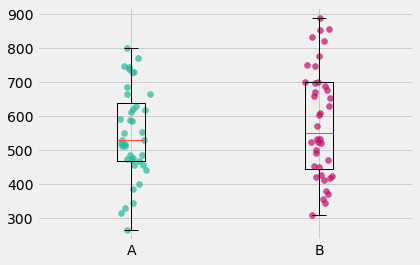# 🗒 Result¶

As a result of the statistical tests applied to the data obtained by the two groups in the research, the groups provided all the necessary statistical assumptions in the literature.

In this direction, it was proved statistically and scientifically that there was no significant difference between the averages of the two groups with 95% confidence.

In line with these results, there is not statistically significant difference between purchase of the control group (Max Bidding) and purchase of the test group (Average Bidding)

# Website Click Through Rate Analysis (CTR)¶

• It is a rate that shows how often users who saw the ad CLICKED the ad.
• Ad Clicks / Ad Shows
In :
control_CTR=control["Click"].sum()/control["Impression"].sum()
test_CTR= test["Click"].sum() / test["Impression"].sum()

print(control_CTR)
print(test_CTR)

0.05014831092596444
0.032922333085396625

In :
df_CTR = pd.DataFrame({"Existing(MAX) CTR":[control_CTR], "New(AVG) CTR": [test_CTR]})
df_CTR.plot.bar(rot=0).legend(loc=3) ;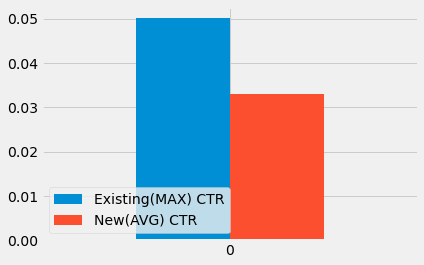Hypothesis
➠Ho: The experiment has no effect on user behavior. (p_cont = p_test)
➠H1: The experiment has an effect on user behavior. (p_cont ≠ p_test)

In :
clicks= np.array([control["Click"].sum(),test["Click"].sum()])
shows= np.array([control["Impression"].sum(), test["Impression"].sum()])
proportions_ztest(count=clicks, nobs=shows)

Out:
(129.3304801715903, 0.0)

# 🗒 Result of CTR¶

p - value < 0.05 ➠ Reject Ho hypthesis. ( The experiment has no effect on user behavior. )

When the bidding methods are examined, the effect of these methods on user behavior (clicks) is different.

And this difference is in favor of the current "max bidding" method.

# Conversion Rate¶

Conversion rate is calculated by dividing the number of conversions by the total number of visitors.

For example, if an ecommerce site gets 200 visitors a month and has 50 sales, the conversion rate would equal 25%.

In :
control_CR = control["Purchase"].sum()/control["Impression"].sum()
test_CR = test["Purchase"].sum() / test["Impression"].sum()

print("Max Bidding CR:", round(control_CR,4))
print("AVG Bidding CR:", round(test_CR,4))

Max Bidding CR: 0.0054
AVG Bidding CR: 0.0048

In :
df_CR=pd.DataFrame({"Existing(max) CR":[control_CR], "New(avg) CR": [test_CR]})
df_CR.plot.bar(rot=0).legend(loc=3) ;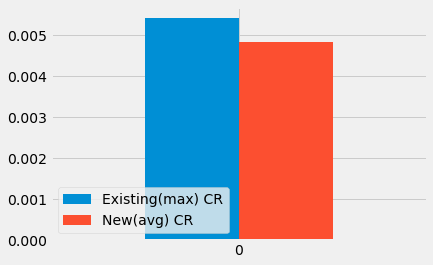Hypothesis
➠Ho: The experiment has no effect on user behavior. (p_cont = p_test)
➠H1: The experiment has an effect on user behavior. (p_cont ≠ p_test)

In :
purchase= np.array([control["Purchase"].sum(),test["Purchase"].sum()])
impression= np.array([control["Impression"].sum(), test["Impression"].sum()])
proportions_ztest(count=purchase, nobs=impression)

Out:
(12.221173465876399, 2.39600929947382e-34)

# 🗒 Result of Conversion Rate¶

p - value < 0.05 ➠ Reject Ho hypthesis. ( The experiment has no effect on user behavior. )

When the bidding methods are examined, the effect of these methods on user behavior (Purchasing) is different.

And this difference is in favor of the current "max bidding" method.